# Modular arithmetic facts for kids

Kids Encyclopedia Facts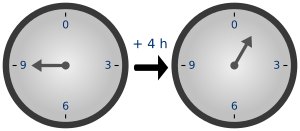Time-keeping on a clock gives an example of modular arithmetic.

Modular arithmetic, sometimes also called clock arithmetic, is a way of doing arithmetic with integers. Much like hours on a clock, which repeat every twelve hours, once the numbers reach a certain value, called the modulus, they go back to zero.

In general, given a modulus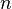$n$, we can do addition, subtraction and multiplication on the set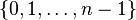$\{ 0, 1, \ldots, n-1\}$ in a way that "wrap around"$n$. This set is sometimes represented by the symbol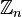$\mathbb{Z}_n$, and called the set of integers modulo$n$.

People talked about modular arithmetic in many ancient cultures. For instance, the Chinese remainder theorem is many centuries old. The modern notation and exact definition of modular arithmetic were first described by Carl Friedrich Gauss.

## Congruence

Modular arithmetic can be used to show the idea of congruence. In general, given a positive integer n, two integers a and b are congruent modulo n, if they have the same remainder when both are divided by n. Congruence can be written this way: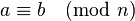$a \equiv b \pmod n\,$

The number n is called the modulus. Another definition of congruence, that means the same thing but is sometimes more useful, is that the two integers are congruent modulo n if the difference (a - b) is an integer multiple of n. That is, if n is a factor of (a - b), then a and b are congruent mod n.

For example: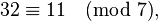$32 \equiv 11 \pmod 7,\,$

The remainder when 32 is divided by 7 is 4, and the remainder when 11 is divided by 7 is also 4. This tells us that they are congruent—when the modulus is 7.

We can use this example with the other definition too. The difference, (a - b), is 32 - 11 = 21. This shows the two numbers are congruent, because 21 = 3 × 7 and 7 is a factor of 21.

## Uses

Modular arithmetic is useful in many fields, and its applications include RSA algorithm in cryptography, primality testing in computer science, and 12-tone arithmetic in music. It is often used in calculating checksums and check digits.

## Related pagesModular arithmetic Facts for Kids. Kiddle Encyclopedia.